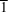International Tables for Crystallography (2006). Vol. C. ch. 9.7, pp. 897-906https://doi.org/10.1107/97809553602060000623

## Contents

• 9.7. The space-group distribution of molecular organic structures  (pp. 897-906)
• 9.7.1. A priori classifications of space groups  (pp. 897-900) | html | pdf |
• 9.7.1.1. Kitajgorodskij's categories  (p. 897) | html | pdf |
• 9.7.1.2. Symmorphism and antimorphism  (pp. 897-899) | html | pdf |
• 9.7.1.3. Comparison of Kitajgorodskij's and Wilson's classifications  (pp. 899-900) | html | pdf |
• 9.7.1.4. Relation to structural classes  (p. 900) | html | pdf |
• 9.7.2. Special positions of given symmetry  (pp. 900-902) | html | pdf |
• 9.7.3. Empirical space-group frequencies  (p. 902) | html | pdf |
• 9.7.4. Use of molecular symmetry  (pp. 902-904) | html | pdf |
• 9.7.4.1. Positions with symmetry 1  (p. 902) | html | pdf |
• 9.7.4.2. Positions with symmetry(pp. 902-903) | html | pdf |
• 9.7.4.3. Other symmetries  (p. 903) | html | pdf |
• 9.7.4.4. Positions with the full symmetry of the geometric class  (pp. 903-904) | html | pdf |
• 9.7.5. Structural classes  (p. 904) | html | pdf |
• 9.7.6. A statistical model  (p. 904) | html | pdf |
• 9.7.7. Molecular packing  (pp. 904-906) | html | pdf |
• 9.7.7.1. Relation to sphere packing  (pp. 904-906) | html | pdf |
• 9.7.7.2. The hydrogen bond and the definition of the packing units  (p. 906) | html | pdf |
• 9.7.8. A priori predictions of molecular crystal structures  (p. 906) | html | pdf |
• References | html | pdf |
• Tables
• Table 9.7.1.1. Kitajgorodskij's categorization of the triclinic, monoclinic and orthorhombic space groups, as modified by Wilson (1993 a )  (p. 898) | html | pdf |
• Table 9.7.1.2. Space groups arranged by arithmetic crystal class and degree of symmorphism (Wilson, 1993 d ), as frequented by homomolecular structures with one molecule in the general position (in superscript numerals; according to Belsky, Zorkaya & Zorky, 1995)  (pp. 899-901) | html | pdf |
• Table 9.7.2.1. Statistics of the use of Wyckoff positions of specified symmetryin the homomolecular organic crystals, based on the data by Belsky, Zorkaya & Zorky (1995)  (p. 903) | html | pdf |
• Table 9.7.4.1. Occurrence of molecules with specified point group in centred symmorphic and other space groups, based on the statistics by Belsky, Zorkaya & Zorky (1995)  (p. 905) | html | pdf |